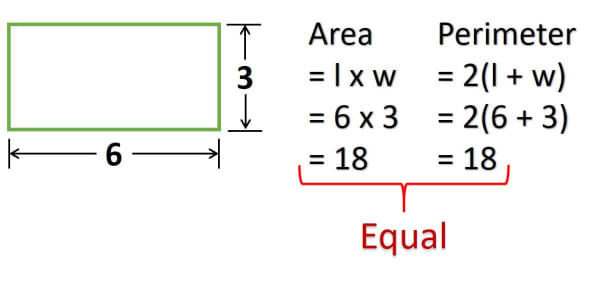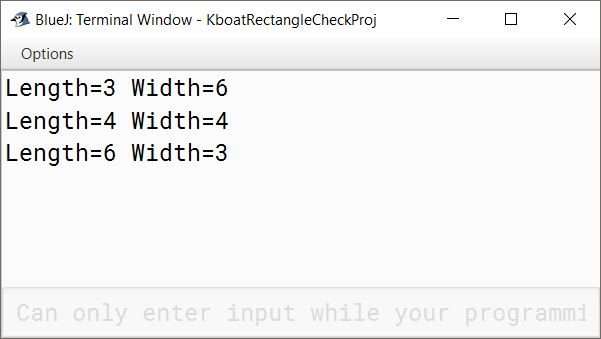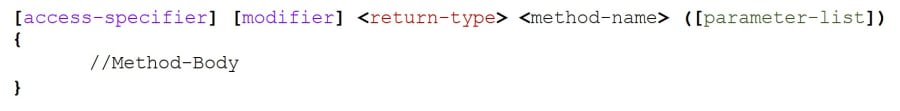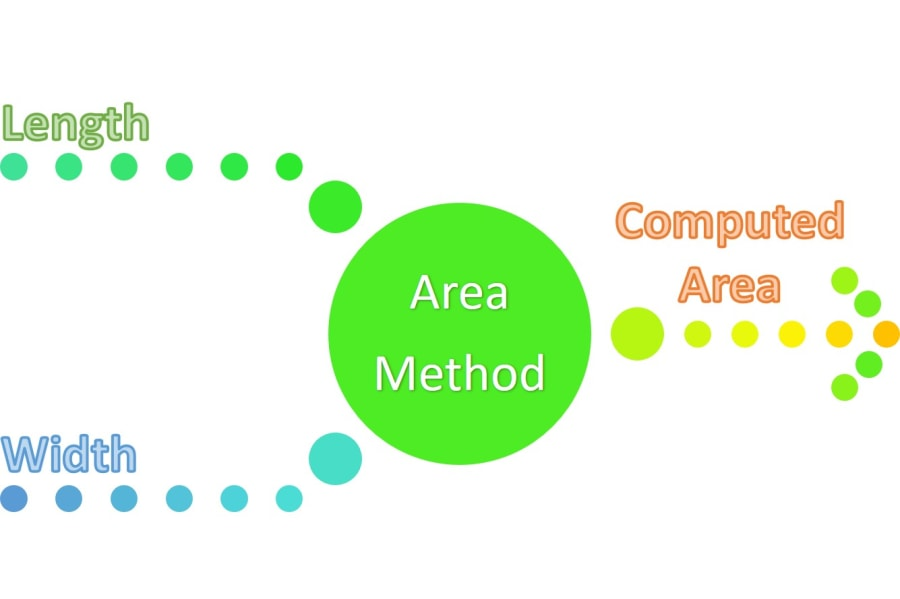# Method Definition - Part 1

## ICSE Computer Applications

In this section we will learn about functions or methods in Java. Functions are an especially important part of programming and almost every programming language supports creating functions.

Over a period of time, different programming languages referred to functions using different names like routines, procedures, functions, method, etc. So, whenever you come across these terms in the programming literature, think of them as some kind of function only.

The term Java uses to refer to functions is Methods. I will use the terms Functions and Methods interchangeably throughout this course. So, don’t get confused, remember that both functions and methods mean the same thing.

## What is a method in Java?

Let’s start by trying to understand what is a method. Often, to solve a problem, we break it down into a set of actions. The solution to the problem involves performing these actions in some sequence. We might have to repeat some of these actions too. Methods help us define these actions in our programs.

### Example

We will look at an example to get more clarity on this. Let’s say we are given a problem to find all the rectangles where area of the rectangle is equal to its perimeter. Simplifying the problem a bit, we need to check for only those rectangles whose sides are integers and are between 1 to 100. Just so you understand this problem clearly, I will quickly show you an example of such a rectangle.The length of this rectangle is 6 and width is 3. Its area and perimeter both are 18. So, we want to write a program to find all such rectangles whose area is equal to its perimeter. To limit our search, we will put a condition on their sides that their sides must be integer values between 1 to 100.

#### Steps to solve the problem

Let’s break down this problem into actions that we need to perform to get the solution:

1. One action here will be, given the length and width of the rectangle we need to find its area.
2. Another action will be to find its perimeter.
3. Next, we compare the area and perimeter and if both are same, we will output the sides of this rectangle

We need to repeat these actions for all lengths between 1 to 100 and all widths between 1 to 100.

#### Pseudo-Code of solution

Let us put these actions in sequence and write a pseudo-code for this:

``````Start a loop from 1 to 100 for length of the rectangle {
Start a loop from 1 to 100 for width of the rectangle {
Compute area;
Compute perimeter;
If (area and perimeter are equal) {
Print length and width of the rectangle
}
}
}
``````

First, I will write this program without using methods. After that I will show you how we can make this program modular using methods and what are the advantages of using methods.

#### Program without methods

```public class KboatRectangleCheckWithoutMethods
{
public static void main(String args[]) {

for (int length = 1; length <= 100; length++) {

for (int width = 1; width <= 100; width++) {

int area = length * width;
int perimeter = 2 * (length + width);

if (area == perimeter) {
System.out.println("Length="
+ length + "\t" + "Width="
+ width);
}

}
}
}
}```

Let's go over the program to understand it. The outer for loop is for length. As we need to check for only integer values of length between 1 to 100 so the loop control variable `length` is of type int and goes from 1 to 100.

For each value of length, we need to compute and compare the area and perimeter of all rectangles having width between 1 to 100. That is, for length 1, we need to find the area and perimeters of all rectangles with width from 1 to 100 similarly for length 2 again we need to find the area and perimeters of all rectangles having width from 1 to 100 and so on. To do this, I have written an inner for loop inside the outer for loop to form a nested loop.

Next, I am calculating the area and the perimeter. After that, I am comparing the area and perimeter using the if statement. If area and perimeter are equal, we have found our rectangle so I am printing its length and width to the console.

#### OutputAs you can see in the output, we found three rectangles whose area and perimeter are equal. The length and width of these three rectangles are (3,6), (4,4) and (6,3), respectively. Effectively there are only two rectangles with sides — (3,6) and (4,4). The third one, (6,3) is same as (3,6) with its length and width swapped.

This is the no methods version of the solution. Now, let’s see how we can introduce methods here.

#### Introducing methods in the program

As I said earlier, the actions required to solve a problem can be defined as methods. In other words, the independent building blocks of our solution should be abstracted out in our code as methods. This last sentence may sound a bit complex to you but don’t worry. We will refactor this solution to use methods and in this process, you will understand the sentence clearly.

Let’s see what are the actions in this solution that are fairly independent. Given the length and width of the rectangle, calculating its area and perimeter are two actions which are independent. So, I will create two new methods for them. But before we do that, lets look at the syntax of defining methods.

### Method Syntax

The general format of defining a method in Java is like this:We will go over each part of this syntax one by one to understand it. The parts of the definition enclosed in square brackets — [ ] are optional whereas the parts enclosed in angle brackets — <> and method body are required.

#### access-specifier

Method definition generally starts with access-specifier. It controls the visibility of the method. Visibility here means whether or not other classes can access the method. It can be public, private or protected. Writing the access-specifier is optional. If we don’t mention an access-specifier, then default access specifier gets assigned to the method. I will leave the discussion of access specifiers at this only for now. We will look at access specifiers in depth when we will discuss classes. In this chapter, either we will not mention the access specifier or use the public access specifier.

#### modifier

After that comes the modifier. This is also optional. Either we will mention the modifier as static or we will not mention the modifier at all. Making a method static means that we can call it without creating the object of its class. Don’t worry if these access-specifier and modifiers are not very clear to you at this point. We will go over both these topics in detail when we will discuss classes.

#### method-name

Like variables and classes, we assign a name to the method too. And like all other names in Java, name of a method is also an identifier, so it must follow all the identifier naming rules. (Identifier naming rules were covered in Code Blocks & Tokens lesson.) We use the method-name to identify and call the method. We can define a method as a set of code that performs some specific task and is referred to by its name. It can be called at any point in the program by using its name.

#### parameter-list

For a method to perform its task, it may need some values as input. Then, it will perform some processing on that input and generate an output. Recall from our example of rectangles with same area and perimeter that we need to create two methods, one for calculating the area and another for calculating the perimeter.If we consider this circle as the method to calculate area, then it will need length and width as the input and give the computed area as the output.

Now that we know about input output of methods, we are ready to look at parameter list. We use the parameter list to provide inputs to the method. A method can take zero or more inputs, so this parameter list is a comma-separated list of variables enclosed within parentheses. The variables which are part of the parameter list are called as the formal parameters or formal arguments of the method. If the method does not take any inputs, then its parameter list is empty which is denoted by empty parentheses.

#### return-type

The return type in the method syntax denotes the data type of the output that the method will return. The return type can be any primitive or composite data type of Java.

For the area and perimeter methods of our example, the return type will be int. Returning a value is optional for methods. A method may or may not return a value. But as you can see in the method syntax, return type is required. Even if the method doesn't return a value still it will have a return type. For such methods that don’t return a value, we use the keyword void as their return type. That is, if the return type of a method is void, it means that the method doesn’t return any value. We will soon see an example of such a method and it will make this even more clear.

#### method-body

The method body contains the set of statements required by the method to complete its task. This set of statements is enclosed within curly braces.

I hope now you are familiar with the syntax of method definition. Don’t worry if you still feel a little uncomfortable with this method syntax. We will go through several examples that will help you develop a fantastic understanding of methods.

Next, we will go back to our example of rectangles with same area and perimeter and continue its refactoring to use methods.